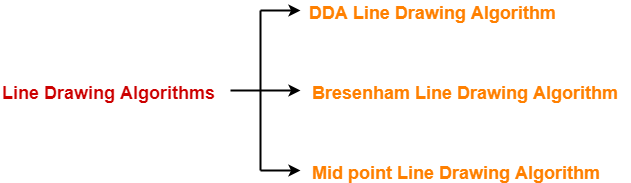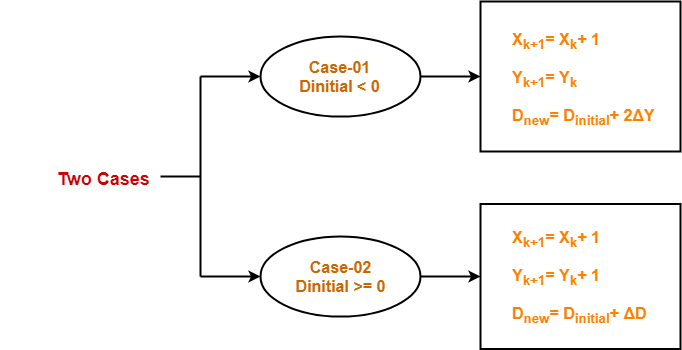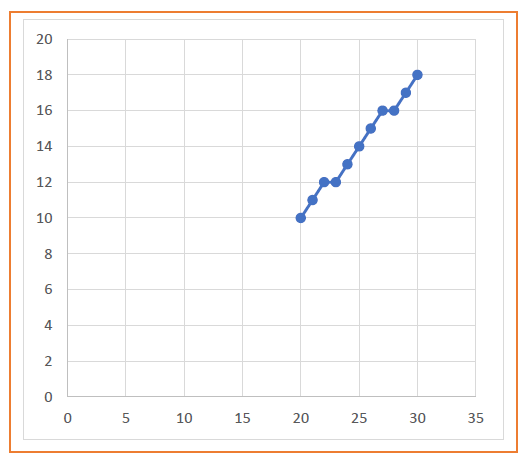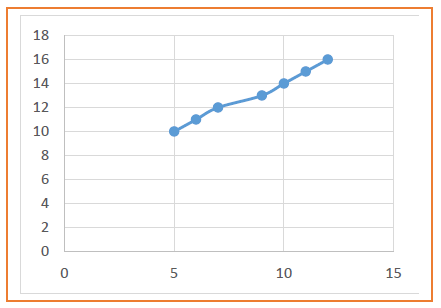# Mid Point Line Drawing Algorithm

## Line Drawing Algorithms-

In computer graphics, popular algorithms used to generate lines are-1. Digital Differential Analyzer (DDA) Line Drawing Algorithm
2. Bresenham Line Drawing Algorithm
3. Mid Point Line Drawing Algorithm

## Mid Point Line Drawing Algorithm-

 Given the starting and ending coordinates of a line, Mid Point Line Drawing Algorithm attempts to generate the points between the starting and ending coordinates.

Also Read- DDA Line Drawing Algorithm

### Procedure-

Given-

• Starting coordinates = (X0, Y0)
• Ending coordinates = (Xn, Yn)

The points generation using Mid Point Line Drawing Algorithm involves the following steps-

### Step-01:

Calculate ΔX and ΔY from the given input.

These parameters are calculated as-

• ΔX = Xn – X0
• ΔY =Yn – Y0

### Step-02:

Calculate the value of initial decision parameter and ΔD.

These parameters are calculated as-

• Dinitial = 2ΔY – ΔX
• ΔD = 2(ΔY – ΔX)

### Step-03:

The decision whether to increment X or Y coordinate depends upon the flowing values of Dinitial.### Step-04:

Keep repeating Step-03 until the end point is reached.

For each Dnew value, follow the above cases to find the next coordinates.

## Problem-01:

Calculate the points between the starting coordinates (20, 10) and ending coordinates (30, 18).

## Solution-

Given-

• Starting coordinates = (X0, Y0) = (20, 10)
• Ending coordinates = (Xn, Yn) = (30, 18)

### Step-01:

Calculate ΔX and ΔY from the given input.

• ΔX = Xn – X0 = 30 – 20 = 10
• ΔY =Yn – Y0 = 18 – 10 = 8

### Step-02:

Calculate Dinitial and ΔD as-

• Dinitial = 2ΔY – ΔX = 2 x 8 – 10 = 6
• ΔD = 2(ΔY – ΔX) = 2 x (8 – 10) = -4

### Step-03:

As Dinitial >= 0, so case-02 is satisfied.

Thus,

• Xk+1 = Xk + 1 = 20 + 1 = 21
• Yk+1 = Yk + 1 = 10 + 1 = 11
• Dnew = Dinitial + ΔD = 6 + (-4) = 2

Similarly, Step-03 is executed until the end point is reached.

 Dinitial Dnew Xk+1 Yk+1 20 10 6 2 21 11 2 -2 22 12 -2 14 23 12 14 10 24 13 10 6 25 14 6 2 26 15 2 -2 27 16 -2 14 28 16 14 10 29 17 10 30 18## Problem-02:

Calculate the points between the starting coordinates (5, 9) and ending coordinates (12, 16).

## Solution-

Given-

• Starting coordinates = (X0, Y0) = (5, 9)
• Ending coordinates = (Xn, Yn) = (12, 16)

### Step-01:

Calculate ΔX and ΔY from the given input.

• ΔX = Xn – X0 = 12 – 5 = 7
• ΔY =Yn – Y0 = 16 – 9 = 7

### Step-02:

Calculate Dinitial and ΔD as-

• Dinitial = 2ΔY – ΔX = 2 x 7 – 7 = 7
• ΔD = 2(ΔY – ΔX) = 2 x (7 – 7) = 0

### Step-03:

As Dinitial >= 0, so case-02 is satisfied.

Thus,

• Xk+1 = Xk + 1 = 5 + 1 = 6
• Yk+1 = Yk + 1 = 9 + 1 = 10
• Dnew = Dinitial + ΔD = 7 + 0 = 7

Similarly, Step-03 is executed until the end point is reached.

 Dinitial Dnew Xk+1 Yk+1 5 9 7 7 6 10 7 7 7 11 7 7 8 12 7 7 9 13 7 7 10 14 7 7 11 15 7 12 16## Advantages of Mid Point Line Drawing Algorithm-

The advantages of Mid Point Line Drawing Algorithm are-

• Accuracy of finding points is a key feature of this algorithm.
• It is simple to implement.
• It uses basic arithmetic operations.
• It takes less time for computation.
• The resulted line is smooth as compared to other line drawing algorithms.

Also Read- Bresenham Line Drawing Algorithm

## Disadvantages of Mid Point Line Drawing Algorithm-

The disadvantages of Mid Point Line Drawing Algorithm are-

• This algorithm may not be an ideal choice for complex graphics and images.
• In terms of accuracy of finding points, improvement is still needed.
• There is no any remarkable improvement made by this algorithm.

To gain better understanding about Mid Point Line Drawing Algorithm,

Watch this Video Lecture

Next Article- Mid Point Circle Drawing Algorithm

Get more notes and other study material of Computer Graphics.

Watch video lectures by visiting our YouTube channel LearnVidFun.

SummaryArticle Name
Mid Point Line Drawing Algorithm
Description
Line Drawing Algorithms- In computer graphics, Mid Point Line Drawing Algorithm is a famous line drawing algorithm. Mid Point Line Drawing Algorithm attempts to generate the points between the starting and ending coordinates.
Author
Publisher Name
Gate Vidyalay
Publisher Logo